# Intermediate Geometry : How to find the volume of a prism

## Example Questions

1 2 3 4 5 6 8 Next →

### Example Question #95 : Prisms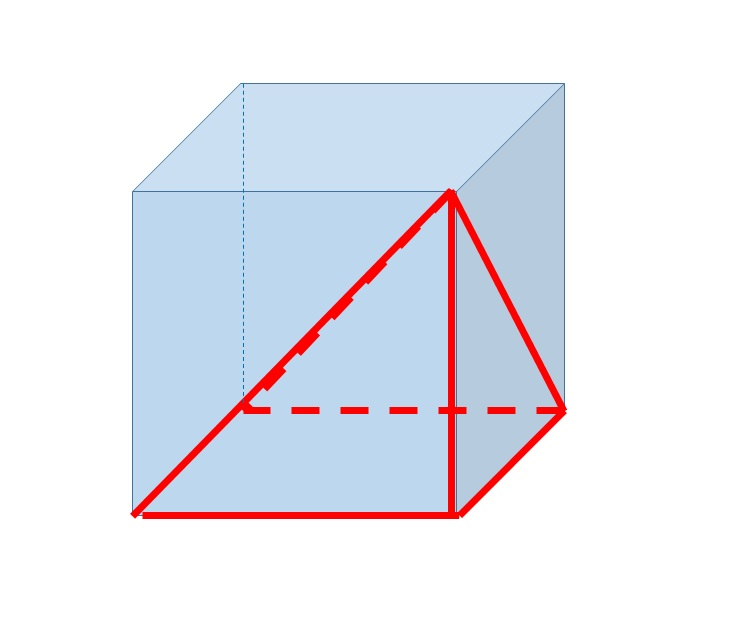The above figure shows a square pyramid inscribed inside a cube. The pyramid has volume 100. Give the volume of the cube.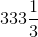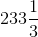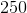Explanation:

Letbe the length of one side of the cube. Then the base of the pyramid is a square with sidelength, and its area is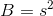.

The volume of a pyramid is one third the product of its height and the area of its base. The height of the pyramid is, so the volume is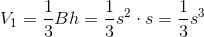The volume of the cube is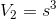, so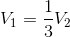or, equivalently,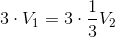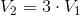That is, the volume of the cube is three times that of the pyramid, and, since the pyramid has volume 100, the volume of the cube is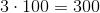.

### Example Question #96 : Prisms

A rectangular box has two sides with the following lengths:andIf it possesses a volume of, what is the area of its largest side?

49

28

21

12

16

28

Explanation:

The volume of a rectangular prism is found using the following formula:If we substitute our known values, then we can solve for the missing side.Divide both sides of the equation by 12.We now know that the missing length equals 7 centimeters.

This means that the box can have sides with the following dimensions: 3cm by 4cm; 7cm by 3cm; or 7cm by 4cm. The greatest area of one side belongs to the one that is 7cm by 4cm.1 2 3 4 5 6 8 Next →